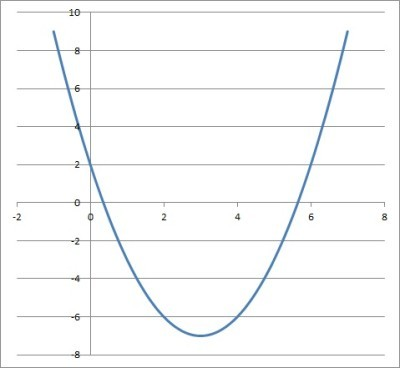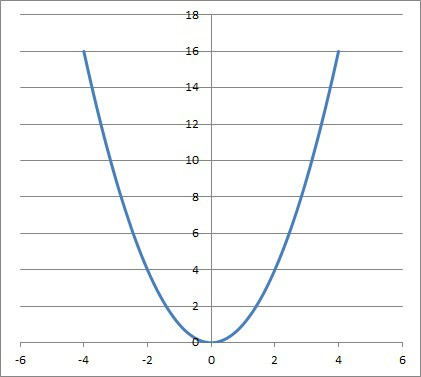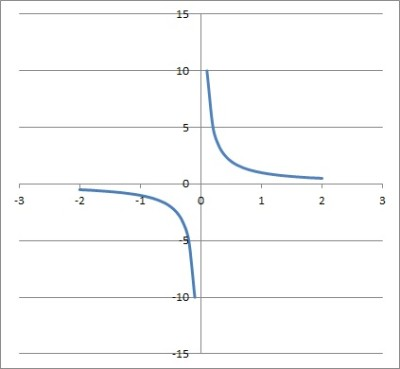# Constants, Variables, & Coefficients (5 Key Things To Know)

Constants, variables, and coefficients are important concepts when we learn algebra.  Although separate ideas, they are related in important ways, and it helps to understand how they are connected.

So, what do you need to know about constants, variables, and coefficients?  A constant is an unchanging number. A variable represents a number that can change. A coefficient is a factor that multiplies a variable, and may contain both constants & variables. Depending on the situation, we may see constants, variables, & coefficients that are negative, fractions, or decimals.

Of course, the values of variables and coefficients may be limited, depending on the context.  For example, if we restrict the domain of a function, it may only have positive values for one of its variables.

Let’s get started.

## Constants, Variables, & Coefficients

Before we get into answering questions about constants, coefficients, and variables, it will help to define each one.

A constant is a number that does not change.  Some examples of constants include:

• π (pi, or 3.14159…, the ratio of a circle’s circumference to its diameter)
• e (Euler’s number, or 2.718…, the base of natural logarithms)
• g (gravity, or acceleration due to gravity on Earth: -9.8 m/s2 or -32 ft/s2).
• 5
• 2.3
• 11/15

These constants are values that do not change, regardless of the situation we are in.

A variable is a symbol that represents a number that may change, depending on the situation we are in.  Some examples of variables include:

• x, the horizontal measure on 2D coordinate axes for graphing functions
• y, the vertical measure on 2D coordinate axes for graphing functions
• p, the position of a falling object
• v, the velocity of a falling object
• a, the acceleration of a falling objectThe variables x and y helps us to graph functions (such as this parabola from a quadratic function) in a 2D coordinate plane.

These variables can take on different values that depend on the situation we are in.

A coefficient is a factor that multiplies a variable in a term or expression.  A coefficient can include constants (numbers), variables, or both.  Some examples of coefficients include:

• the number 5 is the coefficient of the variable x in the term 5x (a constant coefficient)
• the number -7 is the coefficient of the variable y in the term -7y (a constant coefficient)
• the expression 4x is the coefficient of the variable y in the term 4xy
• the expression 4y is the coefficient of the variable x in the term 4xy
• the expression 9xy is the coefficient of the variable z in the term 9xyz

A coefficient can either be constant or take on different values depending on the situation we are in.

### Can A Variable Be Negative?

A variable can be negative in some cases.  This will depend on the domain restriction and on the relationship with other variables.

#### Example 1: A Variable That Is Always Negative

Consider the inequality 2x + 10 < 4.  We can solve as follows:

• 2x < -6
• x < -3

Since x is always less than -3, x is a negative variable.

#### Example 2: A Variable That Is Sometimes Negative

Consider the inequality 3x + 5 < 20.  We can solve as follows:

• 3x < 15
• x < 5

Since x is always less than 5, x is a variable that can be negative, or positive, or zero.

#### Example 3: A Variable That Is Never Negative

Consider the inequality 5x + 6 > 21.  We can solve as follows:

• 5x > 15
• x > 3

Since x is always greater than 3, it is always positive, so x is a variable that cannot be negative.

#### Example 4: A Variable That Is Never Negative

Consider the equation y = x2, where x is a real number.  Since x is real, then x2 will be nonnegative (it is zero is x = 0, and positive otherwise).

That means y is also nonnegative: it is zero when x = 0, and positive otherwise.  So, y is a variable that is never negative.For the quadratic function y = x2, the variable y is negative negative (the graph of the parabola is never below the x-axis).

### Can A Variable Be Zero?

A variable can be zero in some cases.  This will depend on the domain restriction and on the relationship with other variables.

#### Example 1: A Variable That Is Always Zero

Consider the equation 2(x + 3) = 6.  We can solve as follows:

• 2x + 6 = 6
• 2x = 0
• x = 0

So, the variable x can only take on the value zero here.

#### Example 2: A Variable That Is Sometimes Zero

Consider the inequality 6x – 7 < 17.  We can solve as follows:

• 6x < 24
• X < 4

Since x is always less than 4, x is a variable that can be zero, or negative, or positive.

#### Example 3: A Variable That Is Never Zero

Consider the inequality 7x + 2 > 51.  We can solve as follows:

• 7x > 49
• x > 7

Since x is always greater than 7, x is a variable that is always positive: x cannot be zero.

#### Example 4: A Variable That Is Always Zero

Consider the equation y = x2.  If x = 0, then y is automatically zero:

• y = x2
• y = 02
• y = 0

If the variable x is zero here, then the variable y is also zero.

### Can A Variable Be A Term?

A variable can be a term by itself, or it can be a term in an expression.  For example:

• the variable x is a term by itself
• the variable y is a term in the expression 2x + y
• the variable z is a term in the expression 2xy + z
• the variable a is a term in the expression a + b + c
• the variable b is a term in the expression 2a + b – 5c

### Can A Variable Be In The Denominator?

A variable can be in the denominator in some cases.  For example:

• the variable x is in the denominator of the function f(x) = 1/x
• the variable y is in the denominator of the function f(y) = 1/(y – 2)
• the variable z is in the denominator (and also the numerator) of the function f(z) = z/(z – 5)
• the variable a is in the denominator of the function f(x) = b/a
• the variable b is in the denominator of the function f(x) = (a – 2)/(3b – 9)The graph of the function y = 1/x has is undefined for x = 0, since it results in a zero denominator.

Remember that we need to be careful about zero denominators when we find a variable in the denominator of a term.

For example, for the function f(x) = 1/x, a value of x = 0 is undefined (division by zero).

Remember that a variable in the denominator can also be written with a negative exponent.  For example, f(x) = 1/x can also be written as f(x) = x-1.

This means that we cannot have a variable in the denominator for a polynomial.  For a polynomial, all of the exponents for the variables are nonnegative integers.

### Can A Variable Have An Exponent?

A variable can have an exponent, depending on the context.

• For a constant function, the variable can only have an exponent of 0 (constant term).
• For a linear function, the variable can only have an exponent of 1 (linear term) or 0 (constant term).
• For a quadratic function, the variable can only have an exponent of 2 (quadratic term), 1 (linear term) or 0 (constant term).
• For a polynomial function of degree n, the variable in each term can have an integer exponent of any degree from 0 to n.
• A variable can have a rational exponent (fractional power) of a/b, which is equivalent to a radical of the form b√xa.
• In some cases, a variable can have variables in its exponent.  For example, xy or z2x + 3y.

### Can A Variable Be A Coefficient?

A variable can be a coefficient in some cases.  For example:

• The variable y is the coefficient of x in the term xy.
• The variable x is the coefficient of y in the term xy.
• The variable z is the coefficient of t in the term tz.
• The variable t is the coefficient of z in the term tz.

### Can A Coefficient Be Negative?

A coefficient can be negative.  For example:

• The coefficient of the term -2x is -2, a negative number.
• The coefficient of the term -3y is -3, a negative number.
• The coefficient of the variable y in the term -5x2y is -5x2, which is a negative number for x > 0.

### Can A Coefficient Be Zero?

A coefficient can be zero.  However, we do not usually write out these terms to save space and time.  For example:

• For the linear function y = 2x, the constant coefficient is zero: y = 2x + 0.
• For the quadratic function y = x2 + 3, the linear coefficient is zero: y = x2 + 0x + 3.
• For the cubic function y = x3, the quadratic, linear, and constant coefficients are zero: y = x3 + 0x2 + 0x + 0.

### Can A Coefficient Be A Fraction?

A coefficient can be a fraction, as long as we are considering rational coefficients instead of integer ones.  For example:

• For the linear function y = (2/3)x + 5, the linear coefficient is a fraction, 2/3.
• For the function y = (x2 + 3)/ 2, the quadratic coefficient is a fraction, 1/2, and the constant coefficient is a fraction, 3/2.

### Can A Coefficient Be A Decimal?

A coefficient can be a decimal, as long as we are considering rational coefficients instead of integer ones.  For example:

• For the linear function y = 0.6x – 7, the linear coefficient is a decimal, 0.6.
• For the function y = 0.5x2 + 2x + 4, the quadratic coefficient is a decimal, 0.5.

## Conclusion

Now you know the difference between constants, variables, and coefficients.  You also know the answers to some common questions about these terms.